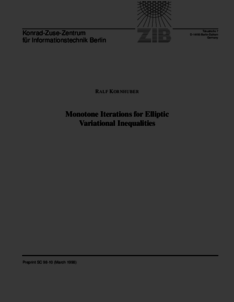Repository: Freie Universität Berlin, Math Department

# Monotone iterations for elliptic variational inequalities

Kornhuber, R. (1999) Monotone iterations for elliptic variational inequalities. In: Free boundary problems: theory and applications. Research notes in mathematics (409). Chapman & Hall/CRC, Boca Raton, London, New York, Washington, D.C., pp. 335-343. ISBN 1-58488-018-XPreview

139kB

## Abstract

A wide range of free boundary problems occurring in engineering and industry can be rewritten as a minimization problem for a strictly convex, piecewise smooth but non–diﬀerentiable energy functional. The fast solution of related discretized problems is a very delicate question, because usual Newton techniques cannot be applied. We propose a new approach based on convex minimization and constrained Newton type linearization. While convex min- imization provides global convergence of the overall iteration, the subsequent constrained Newton type linearization is intended to accelerate the conver- gence speed. We present a general convergence theory and discuss several applications.

Item Type: Book Section Mathematical and Computer Sciences > Mathematics > Numerical Analysis Department of Mathematics and Computer Science > Institute of Mathematics 1909 Ekaterina Engel 20 Jun 2016 20:25 03 Mar 2017 14:42

Repository Staff Only: item control page